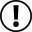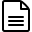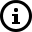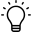# Shock Response of a Composite Overwrapped Pressure VesselA composite overwrapped pressure vessel (COPV) is a tank that holds propellant for space vehicles. The scale of COPV’s can range by a couple orders of magnitude.Throughout a typical mission, these tanks can be subjected to shock/impulse loads derived from launch vibrations and stage separation events.
If a shock load occurs at a frequency near to a natural frequency of the COPV and its mounting structure, then critical peak responses can occur.
Therefore, failure of the COPV and mounting structure must be assessed for shock loads that might occur at varying magnitudes over a range of frequencies.
This loads environment is referred to as a shock response spectrum (SRS).Random vibration analysis is also performed in the design and evaluation of COPV’s, but this article focuses solely on SRS analysis.

# What is a shock response spectrum?

The following excerpt from Nastran's Basic Dynamic Analysis User's Guide provides a good description of SRS analysis:

## 12.4 Response Spectrum Analysis

Response spectrum analysis is an approximate method of computing the peak response of a transient excitation applied to a simple structure or component.

This method is used in civil engineering to predict the peak response of a component on a building that is subjected to earthquake excitation; it is also used in aerospace engineering to predict the peak response of equipment in a spacecraft that is subjected to an impulsive load due to stage separation.

Because it is an approximate method, response spectrum analysis is often used as a design tool. Response spectrum analysis is also called shock spectrum analysis.

There are two parts to response spectrum analysis:
1. generation of the spectrum
2. use of the spectrum for dynamic response such as stress analysis.
Both are available in NX Nastran.Note that the peak response of one oscillator does not necessarily occur at the same time as the peak response for another oscillator. Also, note that there is no phase information since only the magnitude of peak response is computed.It is assumed in this process that each oscillator mass is very small relative to the base structural mass so that the oscillator does not influence the dynamic behavior of the base structure.
Once a spectrum is computed, it can be used for the dynamic response analysis of an NX Nastran model of the component. For example, the spectrum generated for a floor in a building that is subjected to an earthquake can then be applied to a complex model of a piece of equipment attached to that floor. The peak response of each mode of the equipment model is obtained from the spectrum, and those peak model responses are combined to create the overall response. Because the peak responses do not all occur at the same time and only the magnitude of peak responses are computed, various methods are used to combined the peak responses into the overall response.

The combination methods implemented in NX Nastran are SRSS (square root of the sum of the squares), ABS (absolute values), and NRL (U.S. Navy shock design modal summation).The typical response quantities computed are grid point displacements and element stresses.

# Why use a shock response spectrum?The shock response of the system can be evaluated in the frequency domain using spectrum analysis or in the time domain using direct transient analysis.
The chart below provides a summary of the types of dynamic analyses and their corresponding inputs and outputs.While transient analysis can be the most accurate approach when the time domain is finely discretized and the model has many degrees of freedom, it is computationally expensive. It also must be performed multiple times at each frequency having enough effective modal mass to generate significant load.

In contrast, spectrum analysis approximates the peak responses of transient excitations at various frequencies. The peak responses are then combined to generate the maximum expected response across the entire shock spectrum. For these reasons spectrum analysis can be a very useful and efficient design tool.

# Dynamic Analysis of a COPV

## Frequency Response of Approximate Model

A simplified model of the tank and its mounting structure can be used to quite accurately capture the behavior of the system and the shock response.

The model consists of a representative mass element (CONM2) with inertias, an RBE2 element the length of the COPV, and two grounded CBUSH elements with stiffnesses representative of the mounting structure.  The convenience of this model lies in the fact that the stiffnesses of the CBUSH’s can be tuned to achieve the desired behavior and loading.

Once they are tuned, the mounting brackets can then be designed to those target stiffnesses.To understand how the system may respond to the spectrum of shock loads, it is useful to first investigate the natural frequencies of the system and their corresponding mass participation.

The tank has one axial mode (z-direction), which can be derived as follows:The FEA solution yields a natural frequency matching the analytical result and shows a T3 (Z-translation) mass participation factor of 1.0.  This means that 100% of T3 displacement is captured in the first mode and that zero T3 displacement occurs in the other modes.Likewise, the lateral and vertical modes of the tank can be derived as follows:The lateral stiffnesses, k1 and k2, are 75000 lb/in and 85000 lb/in, respectively, and the vertical stiffnesses, k1 and k2, are 350000 lb/in and 90000 lb/in, respectively.

These stiffnesses, according to the derivation above, yield lateral frequencies of 104.93 Hz and 182.45 Hz and vertical frequencies of 131.65 Hz and 323.24 Hz. The FEA solution yields natural frequencies that match the analytical solution.Notice from the figures that the two modes in each direction roughly correspond to a translational mode and a rotational mode.  The lateral direction has a very small amount of coupling between the translational and rotational modes.  Meanwhile, the modes in the vertical direction are much more coupled. The mass participation factors confirm this.The effective modal mass for mass normalized eigenvectors, meffij, is defined by:It follows that for the lateral and vertical directions, which have two modes each:This can be shown by close inspection of the equations of motion.  Furthermore, it indicates that when the two springs have equivalent stiffness, the translational and rotational modes are decoupled.  This coupling behavior is key to understanding the shock response.

## Shock Response Spectrum Analysis Results

Typically, multiple spectra will be provided or derived at various levels of damping.  This allows the SRS analysis to be performed with damping levels that vary with frequency.  In such a case, Nastran will interpolate between the spectra.
In this example, a shock spectrum with 5% damping is provided in log-log scale, and the SRS analysis is performed with a constant 5% damping.The SRS analysis requires what is commonly referred to as base excitation.

An RBE2 element is added to the model connecting the ends of the two CBUSH elements which previously were constrained in the modal analysis.

A mass element is placed at the center of this rigid element and is given a total mass equal to 1e6 times the mass of the tank. This is basically simulating the ground or base as a very heavy mass relative to the tank.

The ground mass is then constrained in all degrees of freedom except the direction of excitation.  In addition, a kinematic (SUPORT) constraint must be defined on the ground mass in the direction of excitation.  The SRS analysis can then be performed in the x-, y-, and z- directions with proper references to the acceleration spectrum and the damping table.

The SRSS combination method, mentioned in the excerpt from the Nastran User’s Guide, was used for these analyses.  The reaction loads at the -Z and +Z ends of the tank are shown in the table below.Unsurprisingly, the vertical reactions have large magnitudes relative to the other reactions.

Recall that the third mode of the system, 131.6 Hz and the fifth mode of the system, 323.2 Hz, have mass participation values of 85% and 15%, respectively. Interpolating the shock spectrum at these frequencies yields g-levels of 45.7 g’s and 226.8 g’s, respectively.  The approximate reaction using the ABS Combination Method yields:This approximation is reasonably close to the 9613 lbs found by the SRS analysis. This approximation serves as a nice illustration of where the load is coming from and why the vertical reactions are elevated.

If the vertical stiffness of the CBUSH at the -Z end of the system is reduced from 350000 lb/in to 85000 lb/in, then the vertical frequencies become 108 Hz and 188 Hz.  Because the stiffnesses at each end are now of similar magnitudes, the modes are mostly uncoupled, and the mass participation at the fifth mode is now negligible. Performing the SRS analysis on the new system results in more reasonable vertical reactions:## Direct Transient Analysis

The shock response can also be simulated using direct transient analysis. This requires the system to be loaded with a half-sine shock wave at a frequency equal to the natural frequency of the system.  To capture the full shock spectrum, this analysis must be performed for each natural frequency that has significant mass participation.

As an example, direct transient analysis is used to determine the vertical (y-direction) shock response corresponding to the 108 Hz mode. The first step is to determine the dynamic amplification factor of the system.  This requires exciting the base or ground node in the y-direction with an enforced displacement load and performing a transient analysis.  The enforced displacement takes the form of a half-sine wave with a magnitude equal to unity and a frequency of 108 Hz.

The transient response of the tank mass and the ground mass are shown in the figure below.The dynamic amplification factor is 1.47.  Log-log interpolation of the shock spectrum at 108 Hz yields a g-level of 32.2 g’s.  The direct transient shock analysis can now be performed by loading the tank mass node with a half-sine wave that has a magnitude of 3097 lbs at a frequency of 108 Hz.The transient response of the vertical reactions at either end of the tank is shown in the figure below.The peak reactions are 2295 lbs at the -Z end of the tank and 2263 lbs at the +Z end of the tank.  This result is in close agreement with the reactions determined by the SRS analysis in the frequency domain.  The SRS analysis results in slightly higher reactions because it is including load contributions from the higher frequency that has a small amount of mass participation.

Authored by:David Cross, Ph.D.
Senior Structures Engineer

Structural Design and Analysis

# Try it for yourself

We have created a tutorial PDF that recaps the information in this article and goes through the steps to run this analysis in Femap with NX Nastran.